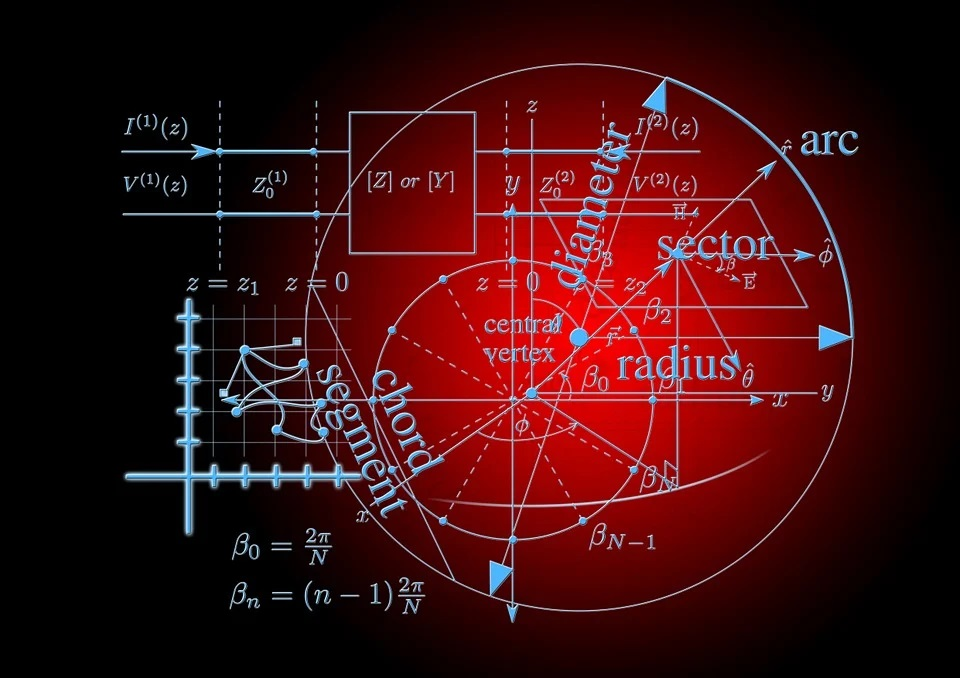## Angles of the Alternate Segment of a Circle Class 10th

Introduction In a circle, if a tangent is drawn then at the point of contact we draw a chord. This chord divides the circle into two segments then the angle made by the chord with the tangent will be equal to the angle made in the alternate segment by the chord. This is the concept …